Share

# HC Verma solutions for Class 12 Concepts of Physics Vol. 2 chapter 16 - Electromagnetic Induction [Latest edition]

Textbook page

#### Chapters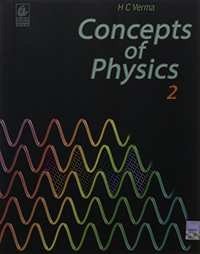## Chapter 16: Electromagnetic Induction

### HC Verma solutions for Class 12 Concepts of Physics Vol. 2 Chapter 16 Electromagnetic Induction Short Answers [Pages 303 - 304]

Short Answers | Q 1 | Page 303

A metallic loop is placed in a nonuniform magnetic field. Will an emf be induced in the loop?

Short Answers | Q 2 | Page 303

An inductor is connected to a battery through a switch. Explain why the emf induced in the inductor is much larger when the switch is opened as compared to the emf induced when the switch is closed.

Short Answers | Q 3 | Page 303

The coil of a moving-coil galvanometer keeps on oscillating for a long time if it is deflected and released. If the ends of the coil are connected together, the oscillation stops at once. Explain.

Short Answers | Q 4 | Page 303

A short magnet is moved along the axis of a conducting loop. Show that the loop repels the magnet if the magnet is approaching the loop and attracts the magnet if it is going away from the loop.

Short Answers | Q 5 | Page 303

Two circular loops are placed coaxially but separated by a distance. A battery is suddenly connected to one of the loops establishing a current in it. Will there be a current induced in the other loop? If yes, when does the current start and when does it end? Do the loops attract each other or do they repel?

Short Answers | Q 6 | Page 303

The battery discussed in the previous question is suddenly disconnected. Is a current induced in the other loop? If yes, when does it start and when does it end? Do the loops attract each other or repel?

Short Answers | Q 7 | Page 303

If the magnetic field outside a copper box is suddenly changed, what happens to the magnetic field inside the box? Such low-resistivity metals are used to form enclosures which shield objects inside them against varying magnetic fields.

Short Answers | Q 8 | Page 303

Metallic (nonferromagnetic) and nonmetallic particles in a solid waste may be separated as follows. The waste is allowed to slide down an incline over permanent magnets. The metallic particles slow down as compared to the nonmetallic ones and hence are separated. Discuss the role of eddy currents in the process.

Short Answers | Q 9 | Page 303

A pivoted aluminium bar falls much more slowly through a small region containing a magnetic field than a similar bar of an insulating material. Explain.

Short Answers | Q 10 | Page 303

A metallic bob A oscillates through the space between the poles of an electromagnet (See the figure). The oscillations are more quickly damped when the circuit is on, as compared to the case when the circuit is off. Explain.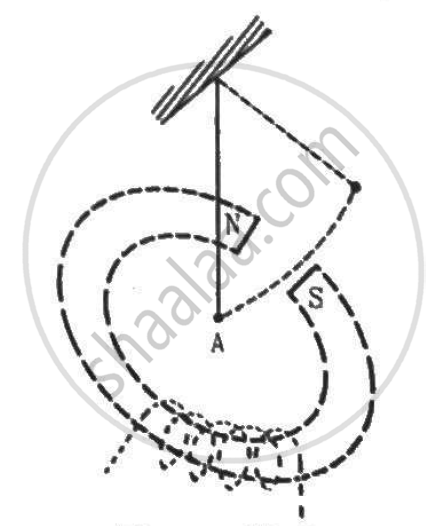Short Answers | Q 11 | Page 304

Two circular loops are placed with their centres separated by a fixed distance. How would you orient the loops to have (a) the largest mutual inductance (b) the smallest mutual inductance?

Short Answers | Q 12 | Page 304

Consider the self-inductance per unit length of a solenoid at its centre and that near its ends. Which of the two is greater?

Short Answers | Q 13 | Page 304

Consider the energy density in a solenoid at its centre and that near its ends. Which of the two is greater?

### HC Verma solutions for Class 12 Concepts of Physics Vol. 2 Chapter 16 Electromagnetic Induction MCQ [Pages 304 - 305]

MCQ | Q 1 | Page 304

A rod of length l rotates with a small but uniform angular velocity ω about its perpendicular bisector. A uniform magnetic field B exists parallel to the axis of rotation. The potential difference between the centre of the rod and an end is ______________ .

• Zero

• 1/8omegaBl^2

• 1/2omegaBl^2

• Bωl2

Q 2 | Page 304

A rod of length l rotates with a uniform angular velocity ω about its perpendicular bisector. A uniform magnetic field B exists parallel to the axis of rotation. The potential difference between the two ends of the rod is ___________ .

• zero

• 1/2Blomega^2

• Blω2

• 2Blω2

MCQ | Q 3 | Page 304

Consider the situation shown in figure. If the switch is closed and after some time it is opened again, the closed loop will show ____________ .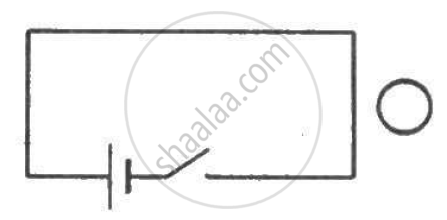• an anticlockwise current-pulse

• a clockwise current-pulse

• an anticlockwise current-pulse and then a clockwise current-pulse

• a clockwise current-pulse and then an anticlockwise current-pulse

Q 4 | Page 304

Consider the situation shown in figure. If the closed loop is completely enclosed in the circuit containing the switch, the closed loop will show _______________ .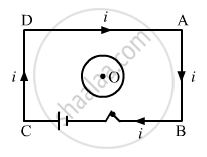• an anticlockwise current-pulse

• a clockwise current-pulse

• an anticlockwise current-pulse and then a clockwise current-pulse

• a clockwise current-pulse and then an anticlockwise current-pulse

MCQ | Q 5 | Page 304

A bar magnet is released from rest along the axis of a very long, vertical copper tube. After some time the magnet ____________ .

• will stop in the tube

• will move with almost contant speed

• will move with an acceleration g

• will oscillate

MCQ | Q 6 | Page 304

Figure shows a horizontal solenoid connected to a battery and a switch. A copper ring is placed on a frictionless track, the axis of the ring being along the axis of the solenoid. As the switch is closed, the ring will __________ .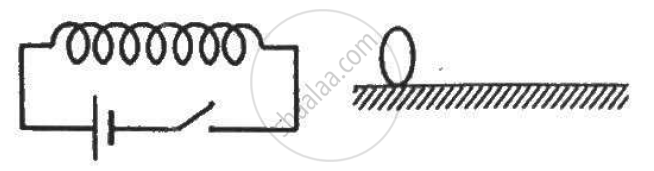• remain stationary

• move towards the solenoid

• move away from the solenoid

• move towards the solenoid or away from it depending on which terminal (positive or negative) of the battery is connected to the left end of the solenoid

MCQ | Q 7 | Page 304

Consider the following statements:-

(A) An emf can be induced by moving a conductor in a magnetic field.
(B) An emf can be induced by changing the magnetic field.

• Both A and B are true

• A is true but B is false

• B is true but A is false

• Both A and B are false

MCQ | Q 8 | Page 304

Consider the situation shown in figure. The wire AB is slid on the fixed rails with a constant velocity. If the wire AB is replaced by a semicircular wire, the magnitude of the induced current will _____________ .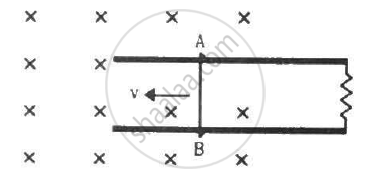• increase

• remain the same

• decrease

• increase or decrease depending on whether the semi-circle bulges towards the resistance or away from it

MCQ | Q 9 | Page 304

Figure shows a conducting loop being pulled out of a magnetic field with a speed v. Which of the four plots shown in figure (b) may represent the power delivered by the pulling agent as a function of the speed v?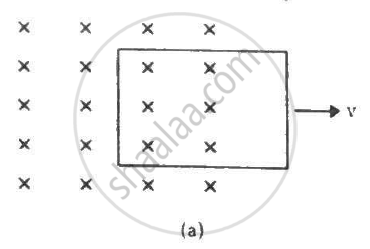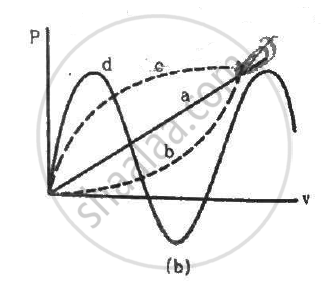MCQ | Q 10 | Page 304

Two circular loops of equal radii are placed coaxially at some separation. The first is cut and a battery is inserted in between to drive a current in it. The current changes slightly because of the variation in resistance with temperature. During this period, the two loops _______________ .

• attract each other

• repel each other

• do not exert any force on each other

• attract or repel each other depending on the sense of the current

MCQ | Q 11 | Page 305

A small, conducting circular loop is placed inside a long solenoid carrying a current. The plane of the loop contains the axis of the solenoid. If the current in the solenoid is varied, the current induced in the loop is __________________ .

• clockwise

• anticlockwise

• zero

• clockwise or anticlockwise depending on whether the resistance is increased or decreased

MCQ | Q 12 | Page 305

A conducting square loop of side l and resistance R moves in its plane with a uniform velocity v perpendicular to one of its sides. A uniform and constant magnetic field Bexists along the perpendicular to the plane of the loop as shown in figure. The current induced in the loop is _____________ .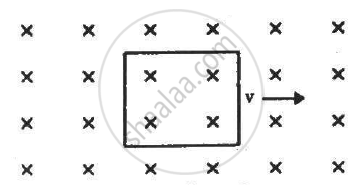• Blv/R clockwise

• Blv/R anticlockwise

• 2Blv/R anticlockwise

• zero

### HC Verma solutions for Class 12 Concepts of Physics Vol. 2 Chapter 16 Electromagnetic Induction MCQ [Page 305]

MCQ | Q 1 | Page 305

A bar magnet is moved along the axis of a copper ring placed far away from the magnet. Looking from the side of the magnet, an anticlockwise current is found to be induced in the ring. Which of the following may be true?
(a) The south pole faces the ring and the magnet moves towards it.
(b) The north pole faces the ring and the magnet moves towards it.
(c) The south pole faces the ring and the magnet moves away from it.
(d) The north pole faces the ring and the magnet moves away from it.

MCQ | Q 2 | Page 305

A conducting rod is moved with a constant velocity v in a magnetic field. A potential difference appears across the two ends _____________ .

• if vec v " || "vec l

• if vec v " || "vec B

• if vec l " || "vec B

• None of these

MCQ | Q 3 | Page 305

A conducting loop is placed in a uniform magnetic field with its plane perpendicular to the field. An emf is induced in the loop if _____________ .
(a) it is translated
(b) it is rotated about its axis
(c) it is rotated about a diameter
(d) it is deformed.

MCQ | Q 4 | Page 305

A metal sheet is placed in front of a strong magnetic pole. A force is needed to ______________ .
(a) hold the sheet there if the metal is magnetic
(b) hold the sheet there if the metal is nonmagnetic
(c) move the sheet away from the pole with uniform velocity if the metal is magnetic
(d) move the sheet away from the pole with uniform velocity if the metal is nonmagnetic.
Neglect any effect of paramagnetism, diamagnetism and gravity.

MCQ | Q 5 | Page 305

A constant current i is maintained in a solenoid. Which of the following quantities will increase if an iron rod is inserted in the solenoid along its axis?
(a) magnetic field at the centre
(b) magnetic flux linked with the solenoid
(c) self-inductance of the solenoid
(d) rate of Joule heating.

MCQ | Q 6 | Page 305

Two solenoids have identical geometrical construction but one is made of thick wire and the other of thin wire. Which of the following quantities are different for the two solenoids?

(a) self-inductance
(b) rate of Joule heating if the same current goes through them
(c) magnetic field energy if the same current goes through them
(d) time constant if one solenoid is connected to one battery and the other is connected to another battery.

MCQ | Q 7 | Page 305

An LR circuit with a battery is connected at t = 0. Which of the following quantities is not zero just after the connection?

• Current in the circuit

• Magnetic field energy in the inductor

• Power delivered by the battery

• Emf induced in the inductor

MCQ | Q 8 | Page 305

A rod AB moves with a uniform velocity v in a uniform magnetic field as shown in figure.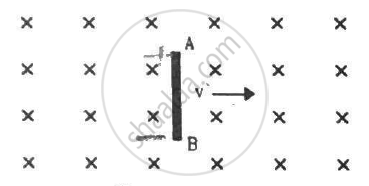• The rod becomes electrically charged

• The end A becomes positively charged

• The end B becomes positively charged.

• The rod becomes hot because of Joule heating

MCQ | Q 9 | Page 305

L, C and R represent the physical quantities inductance, capacitance and resistance respectively. Which of the following combinations have dimensions of frequency?

(a) 1/(RC)

(b) R/L

(c) 1/sqrt(LC)

(d) C/L

MCQ | Q 10 | Page 305

The switches in figure (a) and (b) are closed at t = 0 and reopened after a long time at t = t0.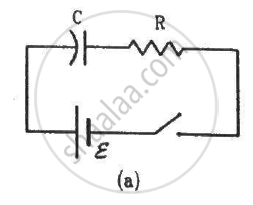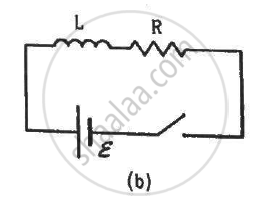(a) The charge on C just after t = 0 is εC.
(b) The charge on C long after t = 0 is εC.
(c) The current in L just before t = t0 is ε/R.
(d) The current in L long after t = t0 is ε/R.

### HC Verma solutions for Class 12 Concepts of Physics Vol. 2 Chapter 16 Electromagnetic Induction [Pages 306 - 313]

Q 1 | Page 306

Calculate the dimensions of (a) $\int \overrightarrow{E} . d \overrightarrow{l,}$ (b) vBl and (c) $\frac{d \Phi_B}{dt}.$ The symbols have their usual meaning.

Q 2 | Page 306

The flux of magnetic field through a closed conducting loop changes with time according to the equation, Φ = at2 + bt + c. (a) Write the SI units of a, b and c. (b) If the magnitudes of a, b and c are 0.20, 0.40 and 0.60 respectively, find the induced emf at t = 2 s.

Q 3 | Page 306

(a) The magnetic field in a region varies as shown in figure. Calculate the average induced emf in a conducting loop of area 2.0 × 10−3 m2 placed perpendicular to the field in each of the 10 ms intervals shown. (b) In which intervals is the emf not constant? Neglect the behaviour near the ends of 10 ms intervals.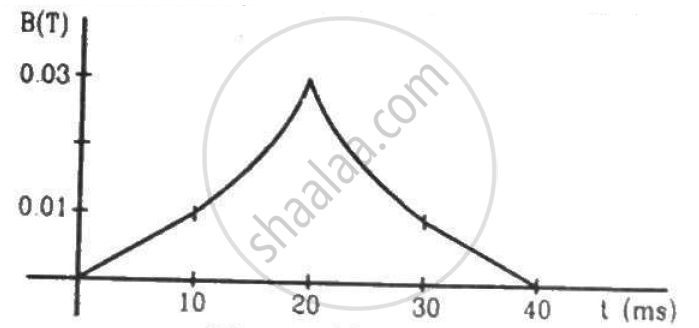Q 4 | Page 306

A conducting circular loop having a radius of 5.0 cm, is placed perpendicular to a magnetic field of 0.50 T. It is removed from the field in 0.50 s. Find the average emf produced in the loop during this time.

Q 5 | Page 306

A conducting circular loop of area 1 mm2 is placed coplanarly with a long, straight wire at a distance of 20 cm from it. The straight wire carries an electric current which changes from 10 A to zero in 0.1 s. Find the average emf induced in the loop in 0.1 s.

Q 6 | Page 306

A square-shaped copper coil has edges of length 50 cm and contains 50 turns. It is placed perpendicular to a 1.0 T magnetic field. It is removed from the magnetic field in 0.25 s and restored in its original place in the next 0.25 s. Find the magnitude of the average emf induced in the loop during (a) its removal, (b) its restoration and (c) its motion.

Q 7 | Page 306

Suppose the resistance of the coil in the previous problem is 25Ω. Assume that the coil moves with uniform velocity during its removal and restoration. Find the thermal energy developed in the coil during (a) its removal, (b) its restoration and (c) its motion.

Q 8 | Page 306

A conducting loop of area 5.0 cm2 is placed in a magnetic field which varies sinusoidally with time as B = B0 sin ωt where B0 = 0.20 T and ω = 300 s−1. The normal to the coil makes an angle of 60° with the field. Find (a) the maximum emf induced in the coil, (b) the emf induced at τ = (π/900)s and (c) the emf induced at t = (π/600) s.

Q 9 | Page 306

Figure shows a conducting square loop placed parallel to the pole-faces of a ring magnet. The pole-faces have an area of 1 cm2 each and the field between the poles is 0.10 T. The wires making the loop are all outside the magnetic field. If the magnet is removed in 1.0 s, what is the average emf induced in the loop?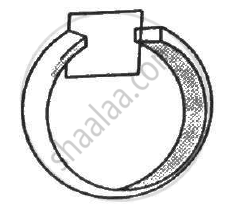Q 10 | Page 306

A conducting square loop having edges of length 2.0 cm is rotated through 180° about a diagonal in 0.20 s. A magnetic field B exists in the region which is perpendicular to the loop in its initial position. If the average induced emf during the rotation is 20 mV, find the magnitude of the magnetic field.

Q 11 | Page 306

A conducting loop of face-area A and resistance R is placed perpendicular to a magnetic field B. The loop is withdrawn completely from the field. Find the charge which flows through any cross-section of the wire in the process. Note that it is independent of the shape of the loop as well as the way it is withdrawn.

Q 12 | Page 306

A long solenoid of radius 2 cm has 100 turns/cm and carries a current of 5 A. A coil of radius 1 cm having 100 turns and a total resistance of 20 Ω is placed inside the solenoid coaxially. The coil is connected to a galvanometer. If the current in the solenoid is reversed in direction, find the charge flown through the galvanometer.

Q 13 | Page 306

Figure shows a metallic square frame of edge a in a vertical plane. A uniform magnetic field B exists in the space in a direction perpendicular to the plane of the figure. Two boys pull the opposite corners of the square to deform it into a rhombus. They start pulling the corners at t = 0 and displace the corners at a uniform speed u. (a) Find the induced emf in the frame at the instant when the angles at these corners reduce to 60°. (b) Find the induced current in the frame at this instant if the total resistance of the frame is  R. (c) Find the total charge which flows through a side of the frame by the time the square is deformed into a straight line.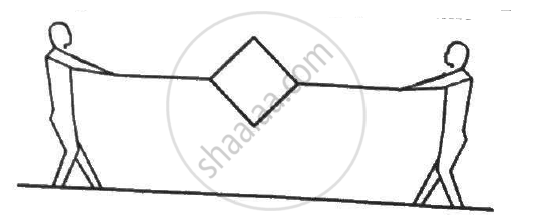Q 14 | Page 307

The north pole of a magnet is brought down along the axis of a horizontal circular coil (see the following figure). As a result, the flux through the coil changes from 0.35 weber to 0.85 weber in an interval of half a second. Find the average emf induced during this period. Is the induced current clockwise or anticlockwise as you look into the coil from the side of the magnet ?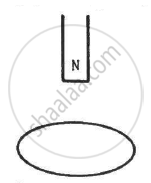Q 15 | Page 307

A wire-loop confined in a plane is rotated in its own plane with some angular velocity. A uniform magnetic field exists in the region. Find the emf induced in the loop.

Q 16 | Page 307

Figure shows a square loop of side 5 cm being moved towards right at a constant speed of 1 cm/s. The front edge enters the 20 cm wide magnetic field at t = 0. Find the emf induced in the loop at (a) t = 2 s, (b) t = 10 s, (c) t = 22 s and (d) t = 30 s.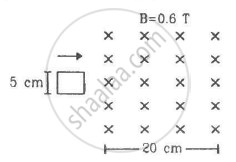Q 17 | Page 307

Figure shows a square loop of side 5 cm being moved towards right at a constant speed of 1 cm/s. The front edge enters the 20 cm wide magnetic field at t = 0. Find the total heat produced in the loop during the interval 0 to 30 s if the resistance of the loop is 4.5 mΩ.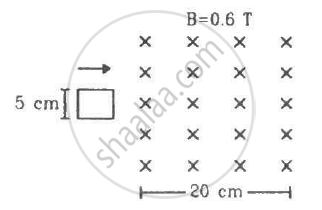Q 18 | Page 307

A uniform magnetic field B exists in a cylindrical region of radius 10 cm as shown in figure. A uniform wire of length 80 cm and resistance 4.0 Ω is bent into a square frame and is placed with one side along a diameter of the cylindrical region. If the magnetic field increases at a constant rate of 0.010 T/s, find the current induced in the frame.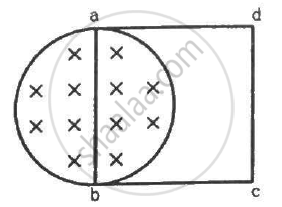Q 19 | Page 307

The magnetic field in the cylindrical region shown in figure increases at a constant rate of 20.0 mT/s. Each side of the square loop abcd and defa has a length of 1.00 cm and a resistance of 4.00 Ω. Find the current (magnitude and sense) in the wire ad if (a) the switch S1 is closed but S2 is open, (b) S1 is open but S2 is closed, (c) both S1 and S2 are open and (d) both S1 and S2 are closed.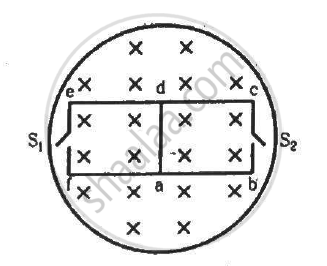Q 20 | Page 307

Figure shows a circular coil of N turns and radius a, connected to a battery of emf εthrough a rheostat. The rheostat has a total length L and resistance R. the resistance of the coil is r. A small circular loop of radius a' and resistance r' is placed coaxially with the coil. The centre of the loop is at a distance x from the centre of the coil. In the beginning, the sliding contact of the rheostat is at the left end and then onwards it is moved towards right at a constant speed v. Find the emf induced in the small circular loop at the instant (a) the contact begins to slide and (b) it has slid through half the length of the rheostat.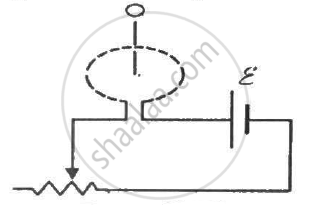Q 21 | Page 307

A circular coil of radius 2.00 cm has 50 turns. A uniform magnetic field B = 0.200 T exists in the space in a direction parallel to the axis of the loop. The coil is now rotated about a diameter through an angle of 60.0°. The operation takes 0.100 s. (a) Find the average emf induced in the coil. (b) If the coil is a closed one (with the two ends joined together) and has a resistance of 4.00 Ω, calculate the net charge crossing a cross-section of the wire of the coil.

Q 22 | Page 307

A closed coil having 100 turns is rotated in a uniform magnetic field B = 4.0 × 10−4 T about a diameter which is perpendicular to the field. The angular velocity of rotation is 300 revolutions per minute. The area of the coil is 25 cm2 and its resistance is 4.0 Ω. Find (a) the average emf developed in half a turn from a position where the coil is perpendicular to the magnetic field, (b) the average emf in a full turn and (c) the net charge displaced in part (a).

Q 23 | Page 307

A coil of radius 10 cm and resistance 40 Ω has 1000 turns. It is placed with its plane vertical and its axis parallel to the magnetic meridian. The coil is connected to a galvanometer and is rotated about the vertical diameter through an angle of 180°. Find the charge which flows through the galvanometer if the horizontal component of the earth's magnetic field is BH = 3.0 × 10−5 T.

Q 24 | Page 307

A circular coil of one turn of radius 5.0 cm is rotated about a diameter with a constant angular speed of 80 revolutions per minute. A uniform magnetic field B = 0.010 T exists in a direction perpendicular to the axis of rotation. Find (a) the maximum emf induced, (b) the average emf induced in the coil over a long period and (c) the average of the squares of emf induced over a long period.

Q 25 | Page 308

A circular coil of one turn of radius 5.0 cm is rotated about a diameter with a constant angular speed of 80 revolutions per minute. A uniform magnetic field B = 0.010 T exists in a direction perpendicular to the axis of rotation. Suppose the ends of the coil are connected to a resistance of 100 Ω. Neglecting the resistance of the coil, find the heat produced in the circuit in one minute.

Q 26 | Page 308

Figure shows a circular wheel of radius 10.0 cm whose upper half, shown dark in the figure, is made of iron and the lower half of wood. The two junctions are joined by an iron rod. A uniform magnetic field B of magnitude 2.00 × 10−4 T exists in the space above the central line as suggested by the figure. The wheel is set into pure rolling on the horizontal surface. If it takes 2.00 seconds for the iron part to come down and the wooden part to go up, find the average emf induced during this period.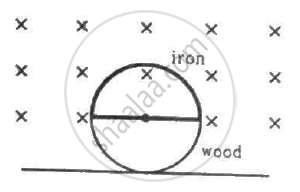Q 27 | Page 308

A 20 cm long conducting rod is set into pure translation with a uniform velocity of 10 cm s−1 perpendicular to its length. A uniform magnetic field of magnitude 0.10 T exists in a direction perpendicular to the plane of motion. (a) Find the average magnetic force on the free electrons of the rod. (b) For what electric field inside the rod, the electric force on a free elctron will balance the magnetic force? How is this electric field created? (c) Find the motional emf between the ends of the rod.

Q 28 | Page 308

A metallic metre stick moves with a velocity of 2 m s−1 in a direction perpendicular to its length and perpendicular to a uniform magnetic field of magnitude 0.2 T. Find the emf induced between the ends of the stick.

Q 29 | Page 308

A 10 m wide spacecraft moves through the interstellar space at a speed 3 × 107 m s−1. A magnetic field B = 3 × 10−10 T exists in the space in a direction perpendicular to the plane of motion. Treating the spacecraft as a conductor, calculate the emf induced across its width.

Q 30 | Page 308

The two rails of a railway track, insulated from each other and from the ground, are connected to a millivoltmeter. What will be the reading of the millivoltmeter when a train travels on the track at a speed of 180 km h−1? The vertical component of earth's magnetic field is 0.2 × 10−4 T and the rails are separated by 1 m.

Q 31 | Page 308

A right-angled triangle abc, made from a metallic wire, moves at a uniform speed v in its plane as shown in figure. A uniform magnetic field B exists in the perpendicular direction. Find the emf induced (a) in the loop abc, (b) in the segment bc, (c) in the segment ac and (d) in the segment ab.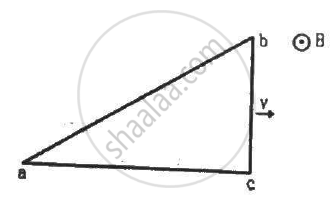Q 32 | Page 308

A copper wire bent in the shape of a semicircle of radius r translates in its plane with a constant velocity v. A uniform magnetic field B exists in the direction perpendicular to the plane of the wire. Find the emf induced between the ends of the wire if (a) the velocity is perpendicular to the diameter joining free ends, (b) the velocity is parallel to this diameter.

Q 33 | Page 308

A wire of length 10 cm translates in a direction making an angle of 60° with its length. The plane of motion is perpendicular to a uniform magnetic field of 1.0 T that exists in the space. Find the emf induced between the ends of the rod if the speed of translation is 20 cm s−1.

Q 34 | Page 308

A circular copper-ring of radius r translates in its plane with a constant velocity v. A uniform magnetic field B exists in the space in a direction perpendicular to the plane of the ring. Consider different pairs of diametrically opposite points on the ring. (a) Between which pair of points is the emf maximum? What is the value of this maximum emf? (b) Between which pair of points is the emf minimum? What is the value of this minimum emf ?

Q 35 | Page 308

Figure shows a wire sliding on two parallel, conducting rails placed at a separation l. A magnetic field B exists in a direction perpendicular to the plane of the rails. What force is necessary to keep the wire moving at a constant velocity v ?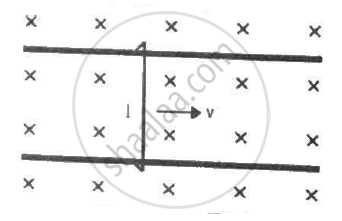Q 36 | Page 308

Figure shows a long U-shaped wire of width l placed in a perpendicular magnetic field B. A wire of length l is slid on the U-shaped wire with a constant velocity v towards right. The resistance of all the wires is r per unit length. At t = 0, the sliding wire is close to the left edge of the U-shaped wire. Draw an equivalent circuit diagram, showing the induced emf as a battery. Calculate the current in the circuit.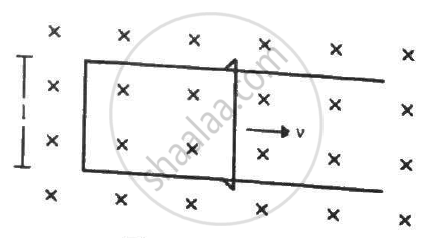Q 37 | Page 308

Figure shows a long U-shaped wire of width l placed in a perpendicular magnetic field B. A wire of length l is slid on the U-shaped wire with a constant velocity v towards right. The resistance of all the wires is r per unit length. At t = 0, the sliding wire is close to the left edge of the U-shaped wire. (a) Calculate the force needed to keep the sliding wire moving with a constant velocity v. (b) If the force needed just after t = 0 is F0, find the time at which the force needed will be F0/2.0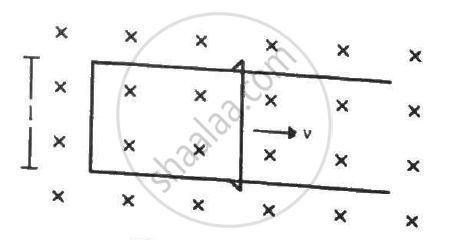Q 38 | Page 309

Consider the situation shown in figure. The wire PQ has mass m, resistance r and can slide on the smooth, horizontal parallel rails separated by a distance l. The resistance of the rails is negligible. A uniform magnetic field B exists in the rectangular region and a resistance R connects the rails outside the field region. At t = 0, the wire PQ is pushed towards right with a speed v0. Find (a) the current in the loop at an instant when the speed of the wire PQ is v, (b) the acceleration of the wire at this instant, (c) the velocity vas a functions of x and (d) the maximum distance the wire will move.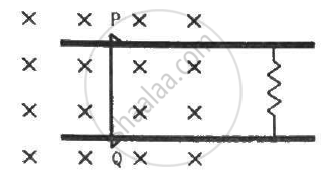Q 39 | Page 309

A rectangular frame of wire abcd has dimensions 32 cm × 8.0 cm and a total resistance of 2.0 Ω. It is pulled out of a magnetic field B = 0.020 T by applying a force of 3.2 × 10−5N (see the following figure). It is found that the frame moves with constant speed. Find (a) this constant speed, (b) the emf induced in the loop, (c) the potential difference between the points aand b and (d) the potential difference between the points c and d.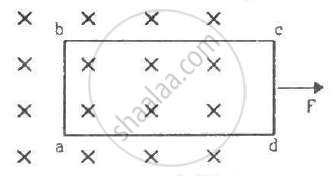Q 40 | Page 309

Figure shows a metallic wire of resistance 0.20 Ω sliding on a horizontal, U-shaped metallic rail. The separation between the parallel arms is 20 cm. An electric current of 2.0 µA passes through the wire when it is slid at a rate of 20 cm s−1. If the horizontal component of the earth's magnetic field is 3.0 × 10−5 T, calculate the dip at the place.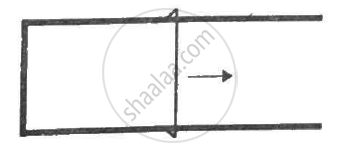Q 41 | Page 309

A wire ab of length l, mass m and resistance R slides on a smooth, thick pair of metallic rails joined at the bottom as shown in figure. The plane of the rails makes an angle θ with the horizontal. A vertical magnetic field B exists in the region. If the wire slides on the rails at a constant speed v, show that $B = \sqrt{\frac{mg R sin\theta}{v l^2 \cos^2 \theta}}$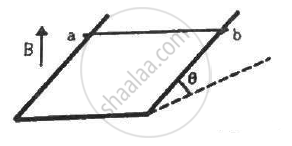Q 42 | Page 309

Consider the situation shown in figure. The wires P1Q1 and P2Q2 are made to slide on the rails with the same speed 5 cm s−1. Find the electric current in the 19 Ω resistor if (a) both the wires move towards right and (b) if P1Q1 moves towards left but P2Q2 moves towards right.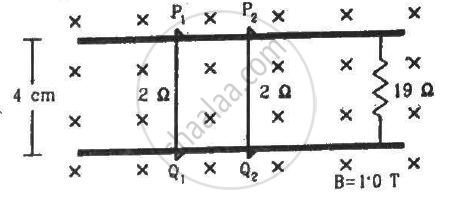Q 43 | Page 309

Consider the situation shown in figure. The wires P1Q1 and P2Q2 are made to slide on the rails with the same speed 5 cm s−1. Suppose the 19 Ω resistor is disconnected. Find the current through P2Q2 if (a) both the wires move towards right and (b) if P1Q1 moves towards left but P2Q2 moves towards right.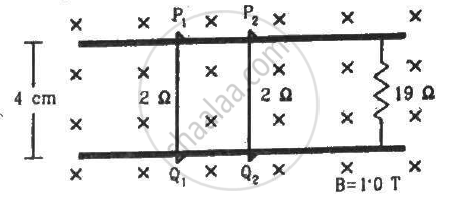Q 44 | Page 309

Consider the situation shown in figure. The wire PQ has a negligible resistance and is made to slide on the three rails with a constant speed of 5 cm s−1. Find the current in the 10 Ω resistor when the switch S is thrown to (a) the middle rail (b) the bottom rail.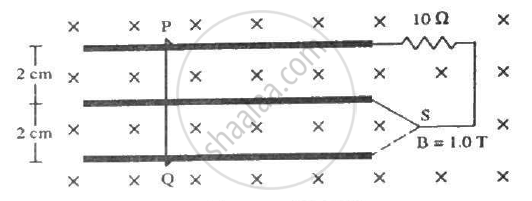Q 45 | Page 309

The current generator Ig' shown in figure, sends a constant current i through the circuit. The wire cd is fixed and ab is made to slide on the smooth, thick rails with a constant velocity v towards right. Each of these wires has resistance r. Find the current through the wire cd.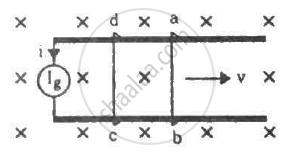Q 46 | Page 309

The current generator Ig' shown in figure, sends a constant current i through the circuit. The wire ab has a length l and mass m and can slide on the smooth, horizontal rails connected to Ig. The entire system lies in a vertical magnetic field B. Find the velocity of the wire as a function of time.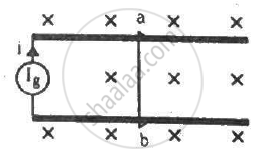Q 47 | Page 309

The current generator Ig' shown in figure, sends a constant current i through the circuit. The wire ab has a length l and mass m and can slide on the smooth, horizontal rails connected to Ig. The entire system lies in a vertical magnetic field B. The system is kept vertically in a uniform horizontal magnetic field B that is perpendicular to the plane of the rails (figure). It is found that the wire stays in equilibrium. If the wire ab is replaced by another wire of double its mass, how long will it take in falling through a distance equal to its length?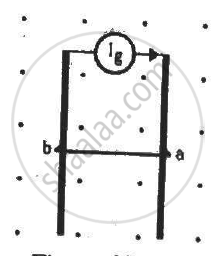Q 48 | Page 310

The rectangular wire-frame, shown in figure, has a width d, mass m, resistance R and a large length. A uniform magnetic field B exists to the left of the frame. A constant force F starts pushing the frame into the magnetic field at t = 0. (a) Find the acceleration of the frame when its speed has increased to v. (b) Show that after some time the frame will move with a constant velocity till the whole frame enters into the magnetic field. Find this velocity v0. (c) Show that the velocity at time t is given by
v = v0(1 − e−Ft/mv0).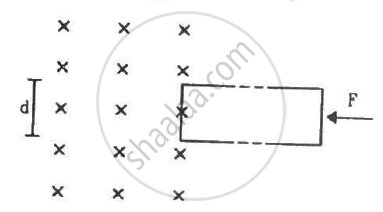Q 49 | Page 310

Figure shows a smooth pair of thick metallic rails connected across a battery of emf εhaving a negligible internal resistance. A wire ab of length l and resistance r can slide smoothly on the rails. The entire system lies in a horizontal plane and is immersed in a uniform vertical magnetic field B. At an instant t, the wire is given a small velocity vtowards right. (a) Find the current in it at this instant. What is the direction of the current? (b) What is the force acting on the wire at this instant? (c) Show that after some time the wire ab will slide with a constant velocity. Find this velocity.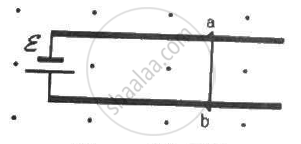Q 50 | Page 310

A conducting wire ab of length l, resistance r and mass m starts sliding at t = 0 down a smooth, vertical, thick pair of connected rails as shown in figure. A uniform magnetic field B exists in the space in a direction perpendicular to the plane of the rails. (a) Write the induced emf in the loop at an instant t when the speed of the wire is v. (b) What would be the magnitude and direction of the induced current in the wire? (c) Find the downward acceleration of the wire at this instant. (d) After sufficient time, the wire starts moving with a constant velocity. Find this velocity vm. (e) Find the velocity of the wire as a function of time. (f) Find the displacement of the wire as a function of time. (g) Show that the rate of heat developed in the wire is equal to the rate at which the gravitational potential energy is decreased after steady state is reached.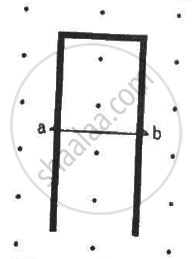Q 51 | Page 309

A bicycle is resting on its stand in the east-west direction and the rear wheel is rotated at an angular speed of 100 revolutions per minute. If the length of each spoke is  30.0 cm and the horizontal component of the earth's magnetic field is 2.0 × 10−5 T, find the emf induced between the axis and the outer end of a spoke. Neglect centripetal force acting on the free electrons of the spoke.

Q 52 | Page 309

A conducting disc of radius r rotates with a small but constant angular velocity ω about its axis. A uniform magnetic field B exists parallel to the axis of rotation. Find the motional emf between the centre and the periphery of the disc.

Q 53 | Page 310

Figure shows a conducting disc rotating about its axis in a perpendicular magnetic field B. A resistor of resistance R is connected between the centre and the rim. Calculate the current in the resistor. Does it enter the disc or leave it at the centre? The radius of the disc is 5.0 cm, angular speed ω = 10 rad/s, B = 0.40 T and R = 10 Ω.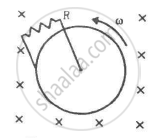Q 54 | Page 310

The magnetic field in a region is given by $\overrightarrow{B} = \overrightarrow{k} \frac{B_0}{L}y$ where L is a fixed length. A conducting rod of length L lies along the Y-axis between the origin and the point (0, L, 0). If the rod moves with a velocity v = v$\overrightarrow{i},$ find the emf induced between the ends of the rod.

Q 55 | Page 310

Figure shows a straight, long wire carrying a current i and a rod of length l coplanar with the wire and perpendicular to it. The rod moves with a constant velocity v in a direction parallel to the wire. The distance of the wire from the centre of the rod is x. Find the motional emf induced in the rod.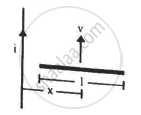Q 56 | Page 311

Consider a situation similar to that of the previous problem except that the ends of the rod slide on a pair of thick metallic rails laid parallel to the wire. At one end the rails are connected by resistor of resistance R. (a) What force is needed to keep the rod sliding at a constant speed v? (b) In this situation what is the current in the resistance R? (c) Find the rate of heat developed in the resistor. (d) Find the power delivered by the external agent exerting the force on the rod.

Q 57 | Page 311

Figure shows a square frame of wire having a total resistance r placed coplanarly with a long, straight wire. The wire carries a current i given by i = i0 sin ωt. Find (a) the flux of the magnetic field through the square frame, (b) the emf induced in the frame and (c) the heat developed in the frame in the time interval 0 to $\frac{20\pi}{\omega}.$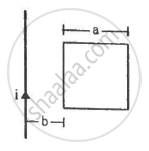Q 58 | Page 311

A rectangular metallic loop of length l and width b is placed coplanarly with a long wire carrying a current i (figure). The loop is moved perpendicular to the wire with a speed vin the plane containing the wire and the loop. Calculate the emf induced in the loop when the rear end of the loop is at a distance a from the wire. solve by using Faraday's law for the flux through the loop and also by replacing different segments with equivalent batteries.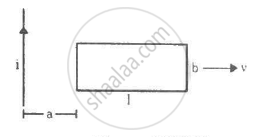Q 59 | Page 311

Figure shows a conducting circular loop of radius a placed in a uniform, perpendicular magnetic field B. A thick metal rod OA is pivoted at the centre O. The other end of the rod touches the loop at A. The centre O and a fixed point C on the loop are connected by a wire OC of resistance R. A force is applied at the middle point of the rod OAperpendicularly, so that the rod rotates clockwise at a uniform angular velocity ω. Find the force.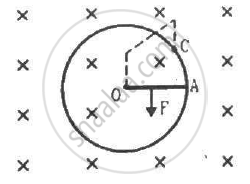Q 60 | Page 311

Consider the situation shown in the figure. Suppose the wire connecting O and C has zero resistance but the circular loop has a resistance Runiformly distributed along its length. The rod OA is made to rotate with a uniform angular speed ω as shown in the figure. Find the current in the rod when ∠ AOC = 90°.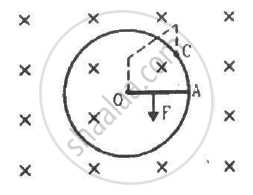Q 61 | Page 311

Consider the situation shown in the figure. Suppose the circular loop lies in a vertical plane. The rod has a mass m. The rod and the loop have negligible resistances but the wire connecting O and C has a resistance R. The rod is made to rotate with a uniform angular velocity ω in the clockwise direction by applying a force at the midpoint of OA in a direction perpendicular to it. Find the magnitude of this force when the rod makes an angle θ with the vertical.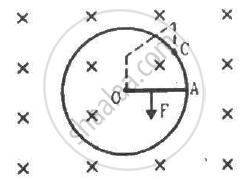Q 62 | Page 311

Suppose the circular loop lies in a vertical plane. The rod has a mass m. The rod and the loop have negligible resistances but the wire connecting O and C has a resistance R. The rod is made to rotate with a uniform angular velocity ω in the clockwise direction by applying a force at the midpoint of OA in a direction perpendicular to it. A battery of emf ε and a variable resistance R are connected between O and C. Neglect the resistance of the connecting wires. Let θ be the angle made by the rod from the horizontal position (show in the figure), measured in the clockwise direction. During the part of the motion 0 < θ < π/4 the only forces acting on the rod are gravity and the forces exerted by the magnetic field and the pivot. However, during the part of the motion, the resistance R is varied in such a way that the rod continues to rotate with a constant angular velocity ω. Find the value of R in terms of the given quantities.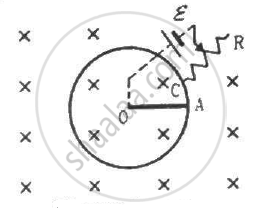Q 63 | Page 311

A wire of mass m and length l can slide freely on a pair of smooth, vertical rails (figure). A magnetic field B exists in the region in the direction perpendicular to the plane of the rails. The rails are connected at the top end by a capacitor of capacitance C. Find the acceleration of the wire neglecting any electric resistance.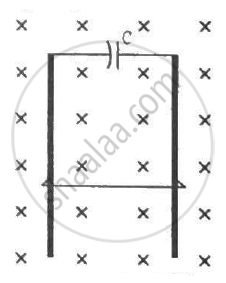Q 64 | Page 311

A uniform magnetic field B exists in a cylindrical region, shown dotted in figure. The magnetic field increases at a constant rate (dB)/(dt). Consider a circle of radius r coaxial with the cylindrical region. (a) Find the magnitude of the electric field E at a point on the circumference of the circle. (b) Consider a point P on the side of the square circumscribing the circle. Show that the component of the induced electric field at P along ba is the same as the magnitude found in part (a).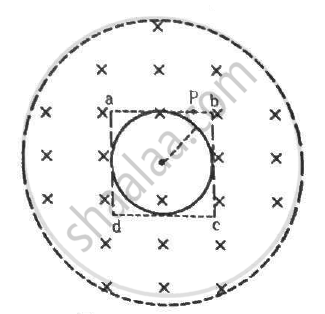Q 65 | Page 312

The current in an ideal, long solenoid is varied at a uniform rate of 0.01 As−1. The solenoid has 2000 turns/m and its radius is 6.0 cm. (a) Consider a circle of radius 1.0 cm inside the solenoid with its axis coinciding with the axis of the solenoid. Write the change in the magnetic flux through this circle in 2.0 seconds. (b) Find the electric field induced at a point on the circumference of the circle. (c) Find the electric field induced at a point outside the solenoid at a distance 8.0 cm from its axis.

Q 66 | Page 312

An average emf of 20 V is induced in an inductor when the current in it is changed from 2.5 A in one direction to the same value in the opposite direction in 0.1 s. Find the self-inductance of the inductor.

Q 67 | Page 312

A magnetic flux of 8 × 10−4 weber is linked with each turn of a 200-turn coil when there is an electric current of 4 A in it. Calculate the self-inductance of the coil.

Q 68 | Page 312

The current in a solenoid of 240 turns, having a length of 12 cm and a radius of 2 cm, changes at a rate of 0.8 A s−1. Find the emf induced in it.

Q 69 | Page 312

Find the value of t/τ for which the current in an LR circuit builds up to (a) 90%, (b) 99% and (c) 99.9% of the steady-state value.

Q 70 | Page 312

An inductor-coil carries a steady-state current of 2.0 A when connected across an ideal battery of emf 4.0 V. If its inductance is 1.0 H, find the time constant of the circuit.

Q 71 | Page 312

A coil having inductance 2.0 H and resistance 20 Ω is connected to a battery of emf 4.0 V. Find (a) the current at the instant 0.20 s after the connection is made and (b) the magnetic field energy at this instant.

Q 72 | Page 312

A coil of resistance 40 Ω is connected across a 4.0 V battery. 0.10 s after the battery is connected, the current in the coil is 63 mA. Find the inductance of the coil.

Q 73 | Page 312

An inductor of inductance 5.0 H, having a negligible resistance, is connected in series with a 100 Ω resistor and a battery of emf 2.0 V. Find the potential difference across the resistor 20 ms after the circuit is switched on.

Q 74 | Page 312

The time constant of an LR circuit is 40 ms. The circuit is connected at t = 0 and the steady-state current is found to be 2.0 A. Find the current at (a) t = 10 ms (b) t = 20 ms, (c) t = 100 ms and (d) t = 1 s.

Q 75 | Page 312

An L-R circuit has L = 1.0 H and R = 20 Ω. It is connected across an emf of 2.0 V at t = 0. Find di/dt at (a) t = 100 ms, (b) t = 200 ms and (c) t = 1.0 s.

Q 76 | Page 312

What are the values of the self-induced emf in the circuit of the previous problem at the times indicated therein?

Q 77 | Page 312

An inductor-coil of inductance 20 mH having resistance 10 Ω is joined to an ideal battery of emf 5.0 V. Find the rate of change of the induced emf at (a) t = 0,  (b) t = 10 ms and (c) t = 1.0 s.

Q 78 | Page 312

An LR circuit contains an inductor of 500 mH, a resistor of 25.0 Ω and an emf of 5.00 V in series. Find the potential difference across the resistor at t = (a) 20.0 ms, (b) 100 ms and (c) 1.00 s.

Q 79 | Page 312

An inductor-coil of resistance 10 Ω and inductance 120 mH is connected across a battery of emf 6 V and internal resistance 2 Ω. Find the charge which flows through the inductor in (a) 10 ms, (b) 20 ms and (c) 100 ms after the connections are made.

Q 80 | Page 312

An inductor-coil of inductance 17 mH is constructed from a copper wire of length 100 m and cross-sectional area 1 mm2. Calculate the time constant of the circuit if this inductor is joined across an ideal battery. The resistivity of copper = 1.7 × 10−8 Ω-m.

Q 81 | Page 312

An LR circuit having a time constant of 50 ms is connected with an ideal battery of emf ε. find the time elapsed before (a) the current reaches half its maximum value, (b) the power dissipated in heat reaches half its maximum value and (c) the magnetic field energy stored in the circuit reaches half its maximum value.

Q 82 | Page 312

A coil having an inductance L and a resistance R is connected to a battery of emf ε. Find the time taken for the magnetic energy stored in the circuit to change from one fourth of the steady-state value to half of the steady-state value.

Q 83 | Page 312

A solenoid having inductance 4.0 H and resistance 10 Ω is connected to a 4.0 V battery at t = 0. Find (a) the time constant, (b) the time elapsed before the current reaches 0.63 of its steady-state value, (c) the power delivered by the battery at this instant and (d) the power dissipated in Joule heating at this instant.

Q 84 | Page 312

The magnetic field at a point inside a 2.0 mH inductor-coil becomes 0.80 of its maximum value in 20 µs when the inductor is joined to a battery. Find the resistance of the circuit.

Q 85 | Page 312

An LR circuit with emf ε is connected at t = 0. (a) Find the charge Q which flows through the battery during 0 to t. (b) Calculate the work done by the battery during this period. (c) Find the heat developed during this period. (d) Find the magnetic field energy stored in the circuit at time t. (e) Verify that the results in the three parts above are consistent with energy conservation.

Q 86 | Page 312

An inductor of inductance 2.00 H is joined in series with a resistor of resistance 200 Ω and a battery of emf 2.00 V. At t = 10 ms, find (a) the current in the circuit, (b) the power delivered by the battery, (c) the power dissipated in heating the resistor and (d) the rate at which energy is being stored in magnetic field.

Q 87 | Page 312

Two coils A and B have inductances 1.0 H and 2.0 H respectively. The resistance of each coil is 10 Ω. Each coil is connected to an ideal battery of emf 2.0 V at t = 0. Let iA and iBbe the currents in the two circuit at time t. Find the ratio iA / iB at (a) t = 100 ms, (b) t = 200 ms and (c) t = 1 s.

Q 88 | Page 312

The current in a discharging LR circuit without the battery drops from 2.0 A to 1.0 A in 0.10 s. (a) Find the time constant of the circuit. (b) If the inductance of the circuit 4.0 H, what is its resistance?

Q 89 | Page 313

A constant current exists in an inductor-coil connected to a battery. The coil is short-circuited and the battery is removed. Show that the charge flown through the coil after the short-circuiting is the same as that which flows in one time constant before the short-circuiting.

Q 90 | Page 313

Consider the circuit shown in figure. (a) Find the current through the battery a long time after the switch S is closed. (b) Suppose the switch is again opened at t = 0. What is the time constant of the discharging circuit? (c) Find the current through the inductor after one time constant.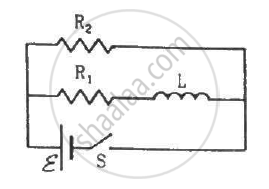Q 91 | Page 313

A current of 1.0 A is established in a tightly wound solenoid of radius 2 cm having 1000 turns/metre. Find the magnetic energy stored in each metre of the solenoid.

Q 92 | Page 313

Consider a small cube of volume 1 mm3 at the centre of a circular loop of radius 10 cm carrying a current of 4 A. Find the magnetic energy stored inside the cube.

Q 93 | Page 313

A long wire carries a current of 4.00 A. Find the energy stored in the magnetic field inside a volume of 1.00 mm3 at a distance of 10.0 cm from the wire.

Q 94 | Page 313

The mutual inductance between two coils is 2.5 H. If the current in one coil is changed at the rate of 1 As−1, what will be the emf induced in the other coil?

Q 95 | Page 313

Find the mutual inductance between the straight wire and the square loop of figure.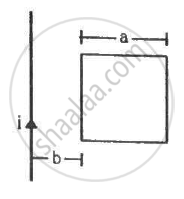Q 96 | Page 313

Find the mutual inductance between the circular coil and the loop shown in figure.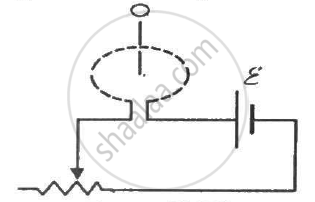Q 97 | Page 313

A solenoid of length 20 cm, area of cross-section 4.0 cm2 and having 4000 turns is placed inside another solenoid of 2000 turns having a cross-sectional area 8.0 cm2 and  length 10 cm. Find the mutual inductance between the solenoids.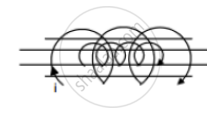Q 98 | Page 313

The current in a long solenoid of radius R and having n turns per unit length is given by i= i0 sin ωt. A coil having N turns is wound around it near the centre. Find (a) the induced emf in the coil and (b) the mutual inductance between the solenoid ant the coil.

## Chapter 16: Electromagnetic Induction## HC Verma solutions for Class 12 Concepts of Physics Vol. 2 chapter 16 - Electromagnetic Induction

HC Verma solutions for Class 12 Concepts of Physics Vol. 2 chapter 16 (Electromagnetic Induction) include all questions with solution and detail explanation. This will clear students doubts about any question and improve application skills while preparing for board exams. The detailed, step-by-step solutions will help you understand the concepts better and clear your confusions, if any. Shaalaa.com has the CBSE Class 12 Concepts of Physics Vol. 2 solutions in a manner that help students grasp basic concepts better and faster.

Further, we at Shaalaa.com provide such solutions so that students can prepare for written exams. HC Verma textbook solutions can be a core help for self-study and acts as a perfect self-help guidance for students.

Concepts covered in Class 12 Concepts of Physics Vol. 2 chapter 16 Electromagnetic Induction are LC Oscillations, Reactance and Impedance, Peak and Rms Value of Alternating Current Or Voltage, Alternating Currents, Power in Ac Circuit: the Power Factor, Ac Voltage Applied to a Series Lcr Circuit, Ac Voltage Applied to an Inductor, Representation of Ac Current and Voltage by Rotating Vectors - Phasors, Ac Voltage Applied to a Resistor, Alternating Currents and Direct Currents, Forced Oscillations and Resonance, Ac Voltage Applied to a Capacitor, Transformers, The Magnetic Dipole Moment of a Revolving Electron, Circular Current Loop as a Magnetic Dipole, Torque on a Rectangular Current Loop in a Uniform Magnetic Field, Magnetic Field on the Axis of a Circular Current Loop, Motion in a Magnetic Field, Velocity Selector, Solenoid and the Toroid - the Toroid, Solenoid and the Toroid - the Solenoid, Magnetic Diapole, Moving Coil Galvanometer, Torque on a Current Loop in Magnetic Field, Force Between Two Parallel Currents, the Ampere, Force on a Current - Carrying Conductor in a Uniform Magnetic Field, Cyclotron, Force on a Moving Charge in Uniform Magnetic and Electric Fields, Straight and Toroidal Solenoids (Only Qualitative Treatment), Ampere’s Circuital Law, Magnetic Field Due to a Current Element, Biot-Savart Law, Oersted’s Experiment, Magnetic Force, Electromagnetic Induction Questions, Ac Generator, Self-Inductance, Energy Consideration: a Quantitative Study, Motional Electromotive Force, The Experiments of Faraday and Henry, Magnetic Flux, Faraday’s Law of Induction, Mutual Inductance, Eddy Currents, Lenz’S Law and Conservation of Energy, Induced Emf and Current, Electromagnetic Induction.

Using HC Verma Class 12 solutions Electromagnetic Induction exercise by students are an easy way to prepare for the exams, as they involve solutions arranged chapter-wise also page wise. The questions involved in HC Verma Solutions are important questions that can be asked in the final exam. Maximum students of CBSE Class 12 prefer HC Verma Textbook Solutions to score more in exam.

Get the free view of chapter 16 Electromagnetic Induction Class 12 extra questions for Class 12 Concepts of Physics Vol. 2 and can use Shaalaa.com to keep it handy for your exam preparation